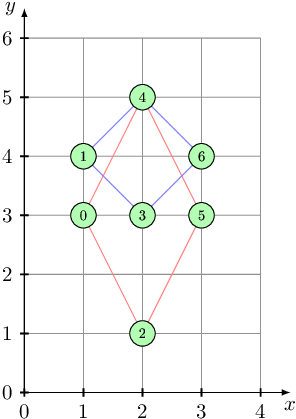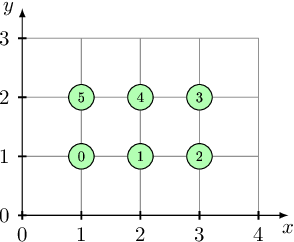Your browser (Unknown 0) is no longer supported. Some parts of the website may not work correctly. Please update your browser.

#### DiamondsCount

Given points on a plane, count the number of sets of four points that form regular diamonds.

A diamond is a quadrilateral whose four sides all have the same length and whose diagonals are parallel to the coordinate axes.

You are given N distinct points on a plane. Count the number of different diamonds that can be constructed using these points as vertices (two diamonds are different if their sets of vertices are different). Do not count diamonds whose area is empty.

Write a function:

class Solution { public int solution(int[] X, int[] Y); }

that, given two arrays X and Y, each containing N integers, representing N points (where X[K], Y[K] are the coordinates of the K-th point), returns the number of diamonds on the plane.

For example, for N = 7 points whose coordinates are specified in arrays X = [1, 1, 2, 2, 2, 3, 3] and Y = [3, 4, 1, 3, 5, 3, 4], the function should return 2, since we can find two diamonds as shown in the picture below:Given arrays: X = [1, 2, 3, 3, 2, 1], Y = [1, 1, 1, 2, 2, 2], the function should return 0, since there are no diamonds on the plane:Write an efficient algorithm for the following assumptions:

• N is an integer within the range [4..1,500];
• each element of arrays X, Y is an integer within the range [0..N−1];
• given N points are pairwise distinct.